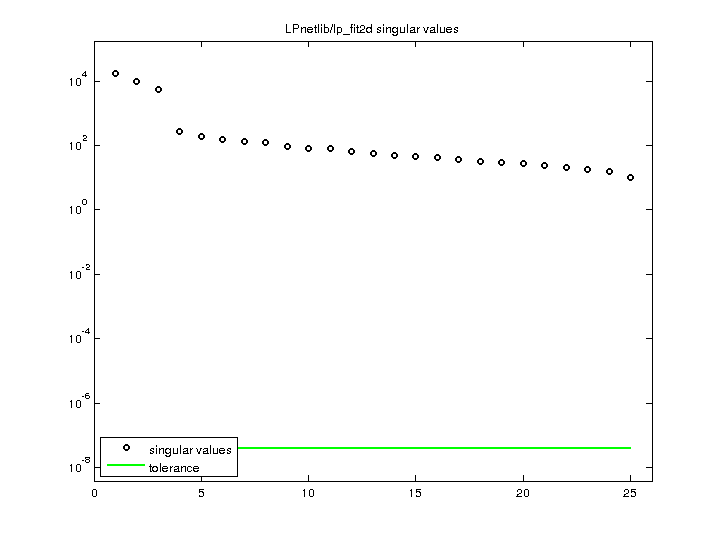Matrix: LPnetlib/lp_fit2d

Description: Netlib LP problem fit2d: minimize c'*x, where Ax=b, lo<=x<=hi(bipartite graph drawing)• Matrix group: LPnetlib
• download as a MATLAB mat-file, file size: 132 KB. Use UFget(626) or UFget('LPnetlib/lp_fit2d') in MATLAB.

 Matrix properties number of rows 25 number of columns 10,524 nonzeros 129,042 structural full rank? yes structural rank 25 # of blocks from dmperm 1 # strongly connected comp. 1 explicit zero entries 0 nonzero pattern symmetry 0% numeric value symmetry 0% type real structure rectangular Cholesky candidate? no positive definite? no

 author R. Fourer editor R. Fourer date 1990 kind linear programming problem 2D/3D problem? no

 Additional fields size and type b full 25-by-1 c full 10524-by-1 lo full 10524-by-1 hi full 10524-by-1 z0 full 1-by-1

Notes:

```A Netlib LP problem, in lp/data.  For more information
send email to netlib@ornl.gov with the message:

send index from lp

The following are relevant excerpts from lp/data/readme (by David M. Gay):

The column and nonzero counts in the PROBLEM SUMMARY TABLE below exclude
slack and surplus columns and the right-hand side vector, but include
the cost row.  We have omitted other free rows and all but the first
right-hand side vector, as noted below.  The byte count is for the
MPS compressed file; it includes a newline character at the end of each
line.  These files start with a blank initial line intended to prevent
mail programs from discarding any of the data.  The BR column indicates
whether a problem has bounds or ranges:  B stands for "has bounds", R
for "has ranges".  The BOUND-TYPE TABLE below shows the bound types
present in those problems that have bounds.

The optimal value is from MINOS version 5.3 (of Sept. 1988)
running on a VAX with default options.

PROBLEM SUMMARY TABLE

Name       Rows   Cols   Nonzeros    Bytes  BR      Optimal Value
FIT2D        26  10500   138018     482330  B    -6.8464293294E+04

BOUND-TYPE TABLE
FIT2D      UP

Supplied by Bob Fourer.
When included in Netlib: Cost coefficients negated.

Concerning FIT1D, FIT1P, FIT2D, FIT2P, Bob Fourer says
The pairs FIT1P/FIT1D and FIT2P/FIT2D are primal and
dual versions of the same two problems [except that we
have negated the cost coefficients of the dual problems
so all are minimization problems].  They originate from
a model for fitting linear inequalities to data, by
minimization of a sum of piecewise-linear penalties.
The FIT1 problems are based on 627 data points and 2-3
pieces per primal pl penalty term.  The FIT2 problems
are based on 3000 data points (from a different sample
altogether) and 4-5 pieces per pl term.

Bob Bixby reports that the CPLEX solver (running on a Sparc station)
finds slightly different optimal values for some of the problems.
On a MIPS processor, MINOS version 5.3 (with crash and scaling of
December 1989) also finds different optimal values for some of the
problems.  The following table shows the values that differ from those
shown above.  (Whether CPLEX finds different values on the recently
added problems remains to be seen.)

Problem        CPLEX(Sparc)          MINOS(MIPS)
FIT2D                            -6.8464293232E+04

Added to Netlib on  31 Jan. 1990
```

 Ordering statistics: result nnz(V) for QR, upper bound nnz(L) for LU, with COLAMD 262,500 nnz(R) for QR, upper bound nnz(U) for LU, with COLAMD 325

 SVD-based statistics: norm(A) 17513.2 min(svd(A)) 10.088 cond(A) 1736.04 rank(A) 25 sprank(A)-rank(A) 0 null space dimension 0 full numerical rank? yes

 singular values (MAT file): click here SVD method used: s = svd (full (R)) ; where [~,R,E] = spqr (A') with droptol of zero status: ok